Back to Kemp Acoustics HomeNext: Lossy propagation Up: Solutions for a cylinder Previous: Solutions for a cylinder   Contents

### Loss-less propagation

Assuming axi-symmetric pressure distributions only, equation (2.31) becomes:(2.44)

which can be manipulated into Bessel's equation of order zero  with the general solution ( p567)(2.45)

where
is Bessel function of the first kind of order zero and
is the Bessel function of the second kind of order zero. Whileis singular at the origin, the pressure cannot have a singularity there. All the physically realisable solutions will therefore have
. The pressure on a cross-section on the-
plane will therefore follow the shape of the Bessel function. A three dimensional plot ofis shown in figure 2.4 while figure 2.5 shows the function along the radial direction. It has a maximum at
and smoothly varies between positive and negative values. The rate at which this happens for
is determined by the-plane wavenumber,
. In order to work out the allowed values of, we must consider the boundary conditions.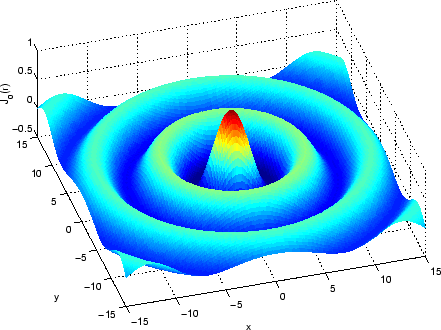The boundary condition for loss-less propagation is(2.46)

Using the orthogonality relation (as used by Kergomard )(2.47)

we get the solution(2.48)

whereare the successive zeros of the derivative of the Bessel function of order zero. From relation (A.3) in Appendix A we see that the derivative ofis
.is therefore also equal to the zeros of the Bessel function of order one. These are tabulated forto
in Appendix A. The corresponding values ofare then:(2.49)

Recalling equation (2.32) the
direction wavenumber is given in terms of the-plane wavenumber and the free space wavenumber as(2.50)

Forthe square root is of a positive number and the positive sign should be taken in equation (2.50) so that
is a positive real wavenumber. For
, however, the square root is of a negative number and the mode will have an imaginary wavenumber in thedirection. Provided the negative sign is chosen in equation (2.50), thedirection pressure profile of that mode will then be(2.51)

which shows exponential damping. The modes of a duct will therefore propagate provided the wavenumber (and therefore frequency) is above the cut-off frequency
and will be exponentially damped otherwise. To summarise, the signs are chosen to be(2.52)

Figure 2.6 displays a false colour image of the forward travelling modes in a cylinder (note one mode is in cut-off so is not really travelling). The horizontal axis runs parallel to the axis of the cylinder () while the vertical axis runs perpendicular to the axis of the cylinder such that the value 0 is the centre of the cylinder and -1 and 1 are the walls. To obtain the pressure distribution along the axis of the cylinder equation (2.52) was substituted into equation (2.33). This was multiplied by the transverse eigenfunction from equation (2.48) to give the full pressure field.

The real value of the complex pressure amplitude was chosen so that a snapshot of the pressure field is shown, rather than a time averaged value, as would be the case if the absolute value was shown. The relationship between the complex pressure amplitude and the time dependence of the pressure field will be discussed in more detail later, in section 2.7. Red indicates the pressure maximum and blue the pressure minimum in each graph.

Figure 2.6 shows plane wave propagation (=0) for a wavenumber of. The=1 mode with the same free space wavenumber is also shown. Notice that the pressure distribution follows the Bessel function profile across a line perpendicular to the cylinder axis. Since the modes we are considering are cylindrically symmetric the two nodal lines parallel to theaxis for=1 become one nodal cylinder when this image is considered in 3 dimensions. The whole distribution varies sinusoidally along theaxis with the wavenumber. Notice that
so thedirection wavenumber has decreased and therefore thedirection wavelength has increased. This effect can be seen more clearly with the=2 mode. The pressure distribution has two nodal cylinders and we can see that
is approaching zero for this choice of free space wavenumber:. The
value is below the cut-off point,
in the case of the=3 mode.meaning that the wave is exponentially damped or evanescent.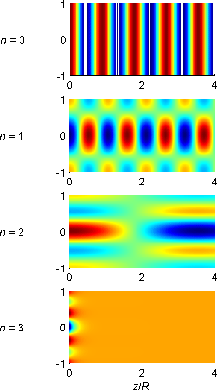Back to Kemp Acoustics HomeNext: Lossy propagation Up: Solutions for a cylinder Previous: Solutions for a cylinder   Contents
Jonathan Kemp 2003-03-24